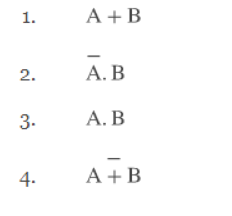A directory of Objective Type Questions covering all the Computer Science subjects. Here you can access and discuss Multiple choice questions and answers for various compitative exams and interviews.

#### Important Notice!

Dear users, compscibits.com is now permanently moved to compsciedu.com. Please update all your links and bookmarks accordingly. Soon, the site will be accessible through URL compsciedu.com only.

 81. The logic expression x’yz’ + x’yz + xyz’ + xyz reduces to: a. x’z b. xyz c. y d. yz

 82. Which of the following binary number is the same as its 2’s complement: a. 1010 b. 0101 c. 1000 d. 1001

 83. A V A = A is called: a. Identity law b. De Morgan’s law c. Idempotent law d. Complement law

 84. An example of a binary number which is equal to its 2’s complement is: a. 1100 b. 1001 c. 1000 d. 1111

 85. Suppose x and y are two Integer Variables having values 0x5AB6 and 0x61CD respectively. The result (in hex) of applying bitwise operator AND to x and y will be: a. 0x5089 b. 0x4084 c. 0x78A4 d. 0x3AD1

 86. 1 Kilo Bytes refers to: a. 1000 Bytes b. 1024 Bytes c. 8000 Bytes d. 8192 Bytes
 87. The following Boolean expression is equivalent toa. 1 b. 2 c. 3 d. 4